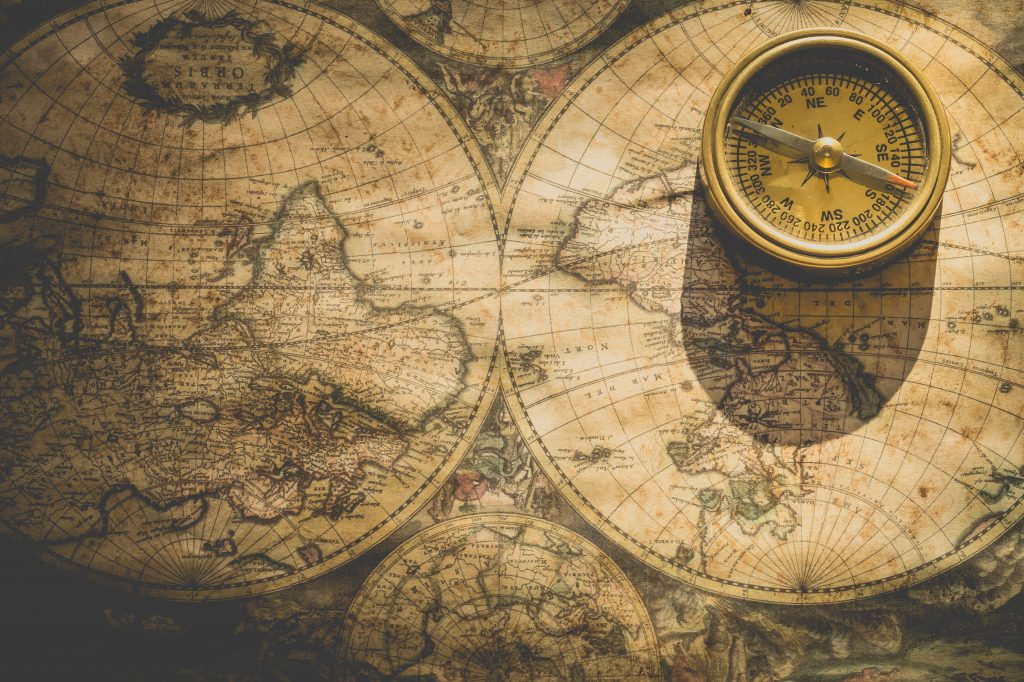Published July 11, 2018As soon as I become more and more observational astronomer, I experience complicated problems such as correcting astrometry of objects in the XMM-Newton field of view. The source positions provided by the telescope are already very good quality and presumably do not differ from actual positions by more than 3.6 arcsec. Nevertheless, there are many cases when the further improvement is desirable. If the Chandra observatory is used the standard package CIAO already has tools to solve this problem, they are reproject_aspect and wcs_match. The standard software of the XMM-Newton (SAS) has the only a tool to recalibrate astrometry based on the optical image obtained by the telescope itself. Nothing is written in manuals about astrometry correction using a list of sources from an external catalog such as the USNO or 2MASS.

It appears that this problem can be solved by means of python and amazing astropy package. The following links were the most useful to understand the principles of WCS (World coordinate system) in FITS (flexible image transport system): old blog post with using pywcsstack exchange post applied to NuStar observations and example of how to use astropy.wcs.

The idea behind the script is quite simple. The astropy library has class wcs which can be initialized directly using the fits header. The wcs object represents a transformation of pixel number on CCD array to right ascension and declination including optical distortions and many more tiny instrumental effects. The information in wcs might be slightly wrong. If you have an astrometric catalog of bright sources, you can improve this information slightly changing two wcs parameters: CRVAL and PC value. First one – is the coordinate reference value and is a vector. The second value is a rotation and is represented by a rotational matrix. After we change both these values we compute new sky coordinate using function all_pix2world. These new coordinates are compared with ones obtained from a catalog and residuals are optimized using e.g. least_squares from scipy.optimize.

Here is an example of the code:

```from scipy.optimize import least_squares
from math import *
import numpy as np
import csv
import pywcs
import pyfits
from astropy.wcs import WCS
import copy
import sys

w = None

## Function which describes a transformation from pixel coordinate to the right ascension and declination

def tranf (param, x_vect):

phi = param ## Rotation angle
A1  = param ## Shift
A2  = param

original_crval = w.wcs.crval.copy() ## Keep original value
original_pc    = w.wcs.pc.copy()    ## here

w.wcs.crval = original_crval.copy() + [A1, A2]
to_matr = [[cos(phi), sin(phi)], [-sin(phi), cos(phi)]] ## The original matrix was identical here, but in principle it should be a matrix multiplication.
w.wcs.pc = to_matr

ra_pred, dec_pred = w.all_pix2world(x_vect, x_vect, 0) ## Compute the sky coordinate. The last value means that we start counting pixels from 0.

w.wcs.crval = original_crval.copy() ## Restore the original values
w.wcs.pc    = original_pc.copy()

return [ra_pred, dec_pred]

## Here we compute residuals between coordinates in catalogue (y) and coordinates derived from the pixel number (x) using parameters of transfromation param
def resid (param, x, y):

res = []
for i in range (0, len(x)):

ra_pred, dec_pred = tranf (param, x[i])
d_ra  = (ra_pred  - y[i]) * 3600  ## The residuals are in arcsec
d_dec = (dec_pred - y[i]) * 3600

#print 'RA, DEC (predicted, actual): ', ra_pred, dec_pred, ' \t ', y[i], y[i]

resid = sqrt(pow(d_ra,2) + pow(d_dec,2.0))
res.append(resid)

return res

x=[]
y=[]
ra=[]
dec=[]

counter = 0

with open('catalog.csv', 'rb') as f:  ## Cross-match between sources detected in my field by the XMM-Newton and USNO B1

if counter == 0:
for i in range (0, len(row)): ## Check that is the header of the table
print i, row[i]
counter = counter + 1

else:

if row != '' and row!= '':
x.append  (float(row)) ## coordinates at the CCD
y.append  (float(row))
ra.append (float(row)) ## coordinates at the sky
dec.append(float(row))

N = len(x)

w = WCS('epn-s.fits') ## Read WCS information from fits file

x0 = [0,  0, 0] ## Initial guess

before_vect = np.zeros((N,2))
after_vect  = np.zeros((N,2))

for i in range (0, N):  ## Initialise the x,y coordinates at CCD and alpha, delta at the sky
before_vect[i] = x[i]
before_vect[i] = y[i]
after_vect[i] = ra[i]
after_vect[i] = dec[i]

res_lsq = least_squares(resid, x0, args=(before_vect, after_vect), max_nfev=30000, method='lm', xtol=1e-15, ftol=1e-15) ## Optimisation

print '--------------'
print res_lsq

final = res_lsq.x

val= resid (final, before_vect, after_vect)

print 'Std: ', np.std(val)  ## Check how good our fit is
```